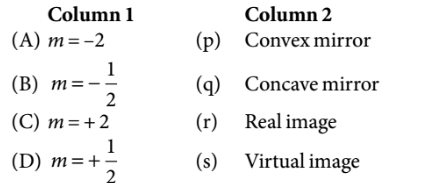Ray optics
Question

# Match the corresponding entries of column 1 with column 2.[Where $m$ is the magnification produced by the mirror]Moderate
Solution

## A) $m=-2⇒v=2u$B) C) $m=+2⇒v=-2u$D) $m=+\frac{1}{2}⇒v=-\frac{u}{2}$As  v and u have different signs with magnification $\left(\frac{1}{2}\right)$ so the mirror is convex and image formed is virtual.

Get Instant Solutions